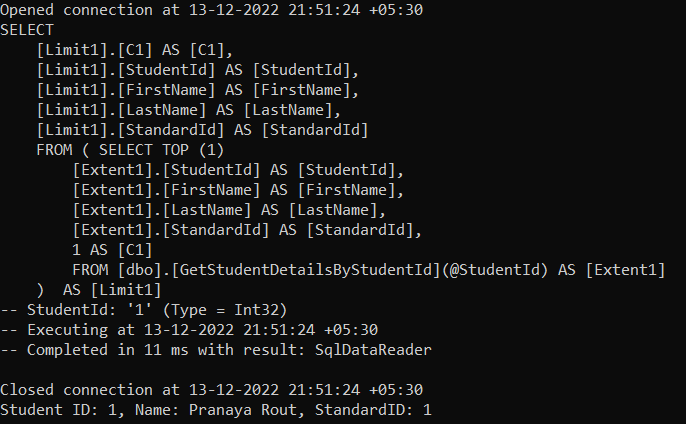# Stored Function in Entity Framework

## Stored Functions in Entity Framework with Examples

In this article, I am going to discuss How to use Stored Functions in Entity Framework Database First Approach with Examples. Please read our previous article where we discussed How to use Stored Procedures in Entity Framework Database First Approach. We are going to work with the same example that we created in our Introduction to Entity Framework Database First Approach article. Please read our introduction to Entity Framework Database First article before proceeding to this article.

##### What is a Function in SQL Server Database?

A function in SQL Server Database is a subprogram or you can say database object that is used to perform an action such as complex calculation and returns the result of the action as a value. There are two types of functions available in SQL Server Database. They are as follows:

1. System Defined Function
2. User-Defined Function

The functions which are already defined by the system and ready to be used by the developer are called System-Defined Functions whereas if the function is defined by the developer or programmer or user then such types of functions are called the user-defined function.

Some functions take parameters; do some processing and return some results back. For example SELECT SQUARE(3)

Some functions may not take any parameters, but returns some result, for example, SELECT GETDATE()

So, we can say that a function can have a parameter(s) that is optional but a function should always return a value that is mandatory.

##### Types of User-Defined Functions in SQL Server:

In SQL Server Database, we can create two types of User-Defined Functions. They are as follows:

##### Scalar Valued User Defined Function in SQL Server:

The user-defined function in SQL Server Database which returns only a single value (i.e. scalar value) is known as the Scalar Valued Function. The Scalar Value Functions in SQL Server may or may not have parameters that are optional but always return a single (scalar) value which is mandatory. The returned value which is returned by the Scalar Function can be of any data type, except text, ntext, image, cursor, and timestamp.

Let us create a user-defined Scaler Value function to return to Student Count. Please execute the below SQL Script on the EF_Demo_DB Database in SQL Server to create the function. Later I will show you how to use this function using Entity Framework.

```-- Scalar Valued User Defined Function to Return the Student Count
CREATE FUNCTION GetStudentCount()
RETURNS INT
AS
BEGIN
DECLARE @StudentCount INT
SET @StudentCount = (SELECT COUNT(*) FROM Student)
RETURN @StudentCount
END
```

To call the above function in SQL Server, use the below statement.

SELECT DBO.GetStudentCount()

##### User-Defined Table-Valued Function in SQL Server

In the case of a Table-Valued Function, we can return a table as an output from the function. These are again of two types as follows.

##### Inline Table-Valued Function in SQL Server:

In the case of the Inline Table-Valued Function in SQL Server, the body of the function will have only a Single Select Statement prepared by the RETURN statement. And here, we need to specify the Return Type as TABLE by using the RETURNS TABLE statement.

Here, we need to specify the TABLE as the Return Type instead of any scalar data type. The function body is not closed between BEGIN and END blocks. This is because the function is going to return a single select statement. The structure of the Table that is going to be returned is determined by the select statement used in the function.

Let us create a user-defined Inline Table-Valued Function to return to Student details by student id. Please execute the below SQL Script on the EF_Demo_DB Database to create the function. Later I will show you how to use this function using Entity Framework.

```-- Inline Table-Valued Function to return Student Details by Student Id
CREATE FUNCTION GetStudentDetailsByStudentId
(
@StudentId INT
)
RETURNS TABLE
AS
RETURN (SELECT * FROM Student WHERE StudentId = @StudentId)
```

To call the above function in SQL Server, use the below statement.

SELECT * FROM GetStudentDetailsByStudentId(1)

##### Multi-Statement Table-Valued Function in SQL Server

The Multi-Statement Table Valued Function in SQL Server is the same as the Inline Table-Valued Function means it is also going to return a table as an output but with the following differences.

1. The Multi-Statement Table-Valued Function body can contain more than one statement. In Inline Table-Valued Function, it contains only a single Select statement prepared by the return statement.
2. In Multi-Statement Table-Valued Function, the structure of the table returned from the function is defined by us. But, in Inline Table-Valued Function, the structure of the table is defined by the Select statement that is going to return from the function body.

Let us create a user-defined Multi-Statement Table-Valued Function to return all Student details. Please execute the below SQL Script on the EF_Demo_DB Database to create the function. Later I will show you how to use this function using Entity Framework.

```-- Multi-statement Table Valued function to return the student details
CREATE FUNCTION GetAllStudentDetails()
RETURNS @StudentTable Table (StudentId INT, Name NVARCHAR(100), StudentAddress NVARCHAR(100))
AS
BEGIN
INSERT INTO @StudentTable
FROM Student S
ON S.StudentId = A.StudentId
Return
End
```

To call the above function in SQL Server, use the below statement.

SELECT * FROM GetAllStudentDetails()

##### How to use Stored Functions with Entity Framework Database First Approach?

We have created three stored functions in our database. Let us proceed and try to understand how to use the above three functions using Entity Framework Database First Approach. Let us first include these stored functions in our Entity Data Model. To do so, open the Visual Designer of Entity Data Model and then right-click and select the Update Model from the Database option from the context menu as shown in the below image.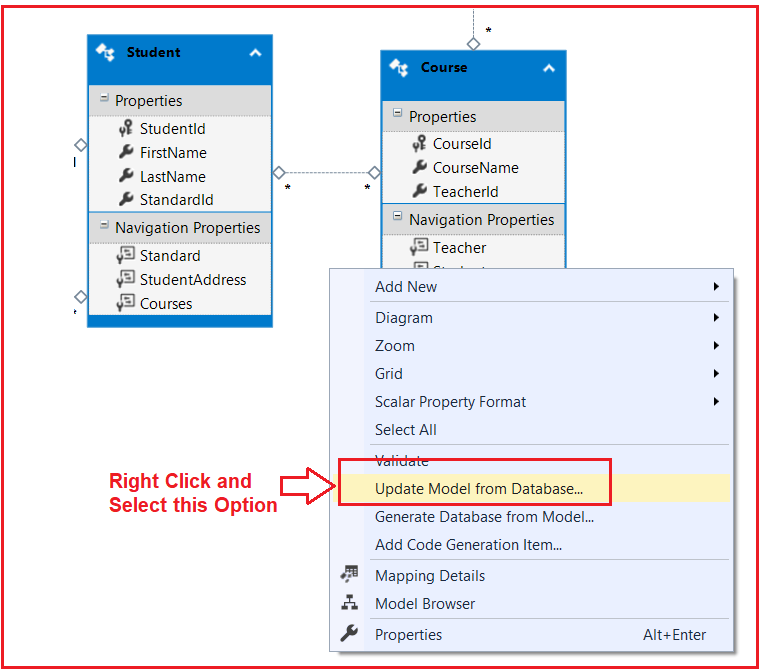This will open the following Update Wizard. From this wizard, expand the Stored Procedure and Functions section from the Add tab and then checked all the checkboxes for all three stored functions, and then click on the Finish button as shown in the below image.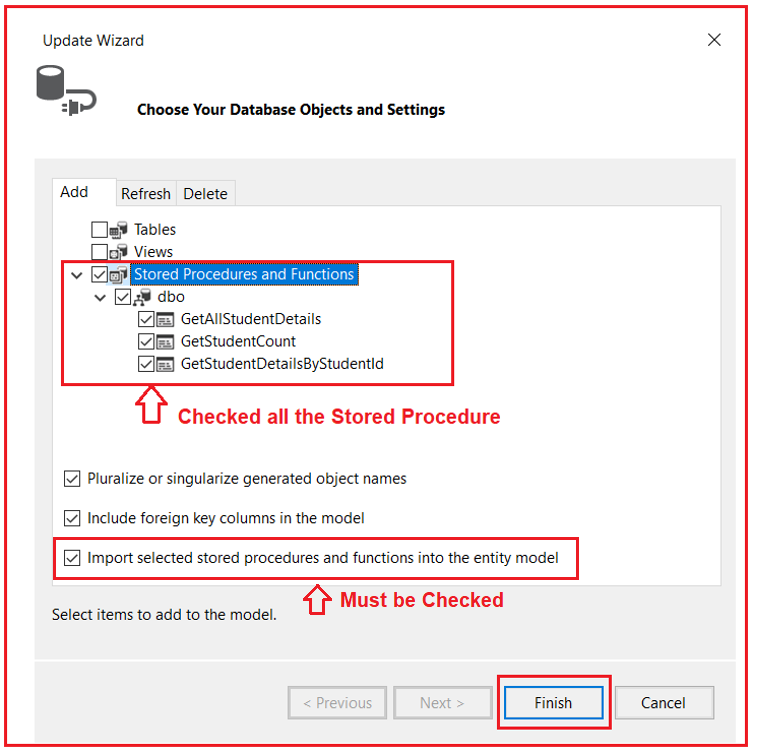Once you click on the Finish button then save the changes in Entity Data Model. Once you save the changes, it will generate two functions within the context class as shown below. The point that you need to remember like the stored procedure, for stored function also it is going to generate methods within the context class.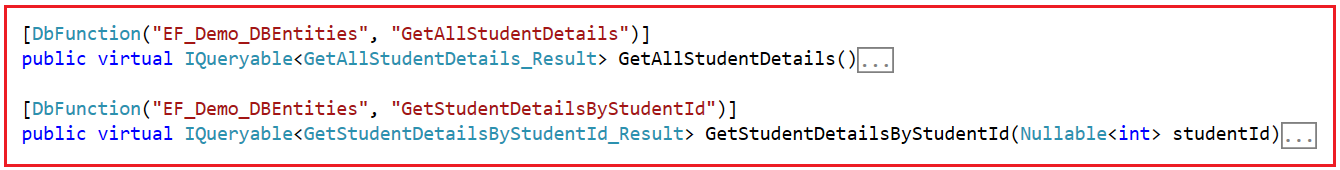Why two Functions? The point that you need to remember the entity framework supports only Table Valued Functions. Here, GetStudentCount is Scalar Valued Function, so the entity framework will not generate the corresponding method in the context class.

Whenever we use the Table Valued Function, for the result, the Entity Framework going to create a complex type based on the table structure of the function. So, in this case, it is going to create two complex types to store the result of the two table-valued functions.

The store result of the GetAllStudentDetails stored function, it created the following GetAllStudentDetails_Result complex type.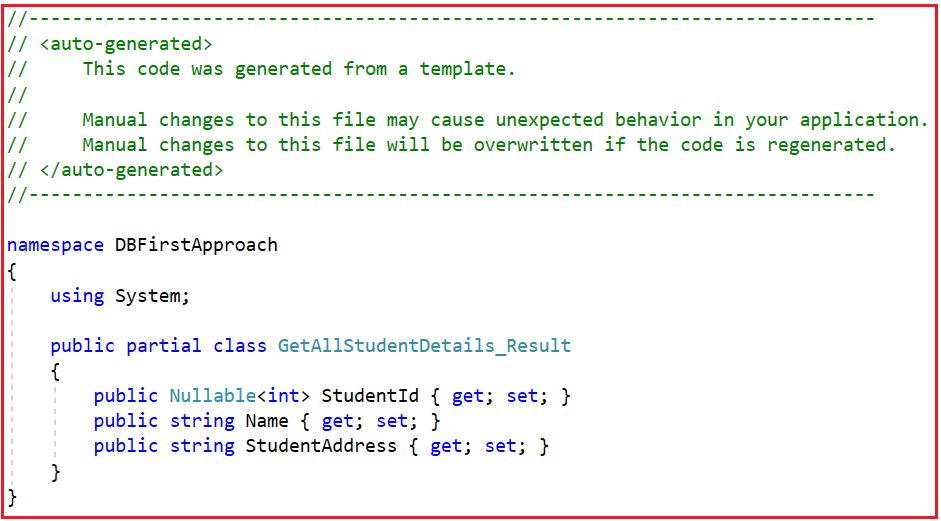Similar to storing the result of the GetStudentDetailsByStudentId stored function it is creating the following GetStudentDetailsByStudentId_Result complex type.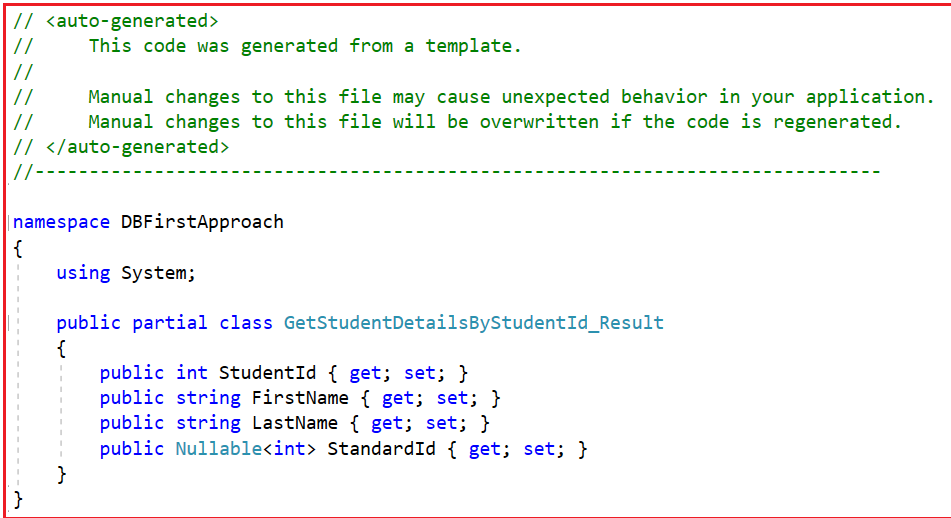##### Calling GetAllStudentDetails Stored Function using Entity Framework:

For GetAllStudentDetails Stored Function, the Entity Framework created the following GetAllStudentDetails method within the context class. This method does not take any parameter and it is going to return the GetAllStudentDetails_Result collection.

```[DbFunction("EF_Demo_DBEntities", "GetAllStudentDetails")]
public virtual IQueryable<GetAllStudentDetails_Result> GetAllStudentDetails()
{
}
```

Now, modify the Main method as shown below. Here, using the context object we are calling the GetAllStudentDetails method which is going to return all the students from the Student database table.

```using System;
namespace DBFirstApproach
{
class Program
{
static void Main(string[] args)
{
using (var context = new EF_Demo_DBEntities())
{
//To See the Generated SQL By Context Object
context.Database.Log = Console.Write;

var Result = context.GetAllStudentDetails();
foreach (var student in Result)
{
}
}
}
}
}
```
###### Output: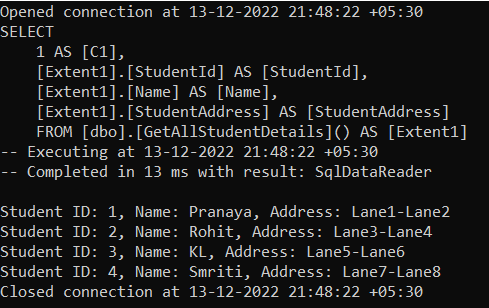##### Calling GetStudentDetailsByStudentId Stored Function using Entity Framework:

For GetStudentDetailsByStudentId Stored Function, the Entity Framework created the following GetStudentDetailsByStudentId method within the context class. This method does take the student id as a parameter and it is going to return the GetStudentDetailsByStudentId_Result collection.

```[DbFunction("EF_Demo_DBEntities", "GetStudentDetailsByStudentId")]
public virtual IQueryable<GetStudentDetailsByStudentId_Result> GetStudentDetailsByStudentId(Nullable<int> studentId)
{
var studentIdParameter = studentId.HasValue ?
new ObjectParameter("StudentId", studentId) :
new ObjectParameter("StudentId", typeof(int));

}
```

Now, modify the Main method as shown below. Here, using the context object we are calling the GetStudentDetailsByStudentId method by passing the Student Id 1 which is going to return the student’s information from the Student database table.

```using System;
using System.Linq;
namespace DBFirstApproach
{
class Program
{
static void Main(string[] args)
{
using (var context = new EF_Demo_DBEntities())
{
//To See the Generated SQL By Context Object
context.Database.Log = Console.Write;

var student = context.GetStudentDetailsByStudentId(1).FirstOrDefault();

Console.WriteLine(\$"Student ID: {student.StudentId}, Name: {student.FirstName} {student.LastName}, StandardID: {student.StandardId}");

}